# Graph Introduction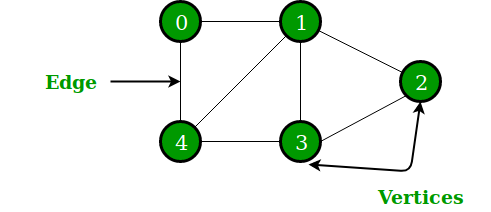Graph Diagram — GeeksForGeeks

Do you mean the graphs they teach you in school?

Well, if you mean the graphs they teach you in mathematics like a bar graph, not necessarily. I mean graphs in computer science terms.

“A graph data structure consists of a finite (and possibly mutable) set of vertices (also called nodes or points), together with a set of unordered pairs of these vertices for an undirected graph or a set of ordered pairs for a directed graph. These pairs are known as edges (also called links or lines), and for a directed graph are also known as arrows.” — Wikipedia

# Directed Graphs

A directed graph is an ordered pair: G = (V, E)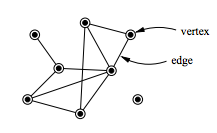Vertices & Edges — Wikipedia

V is a set of vertices — also known as nodes or points

E is a set of edges — , a set of edges (also called directed edges, directed links, directed lines, arrows or arcs) which are ordered pairs of vertices (that is, an edge is associated with two distinct vertices). — Wikipedia

Directed graphs (also known as a Digraph) have edges with directions. The edges indicate a one-way relationship, meaning that each edge is only traversed in a single direction.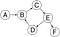Directed Graph — Wikipedia

For example, the edges (b,c) is the edge from node b to node c. For an analogy, you can think of node b driving to node c’s house.

# Undirected Graphs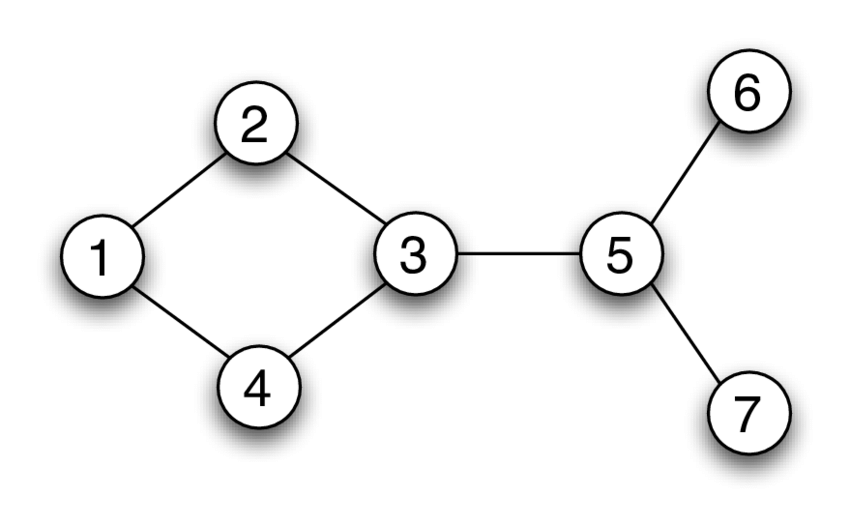Undirected Graph- ResearchGate

An undirected graph is when the edges have no orientation. From the diagram on the left, edge (3,5) can be identical to the edge (5,3).

You can think of an undirected graph like a city, where the vertices (nodes) are the city streets, and the edges (lines) are the bidirectional roads.

# Adjacency List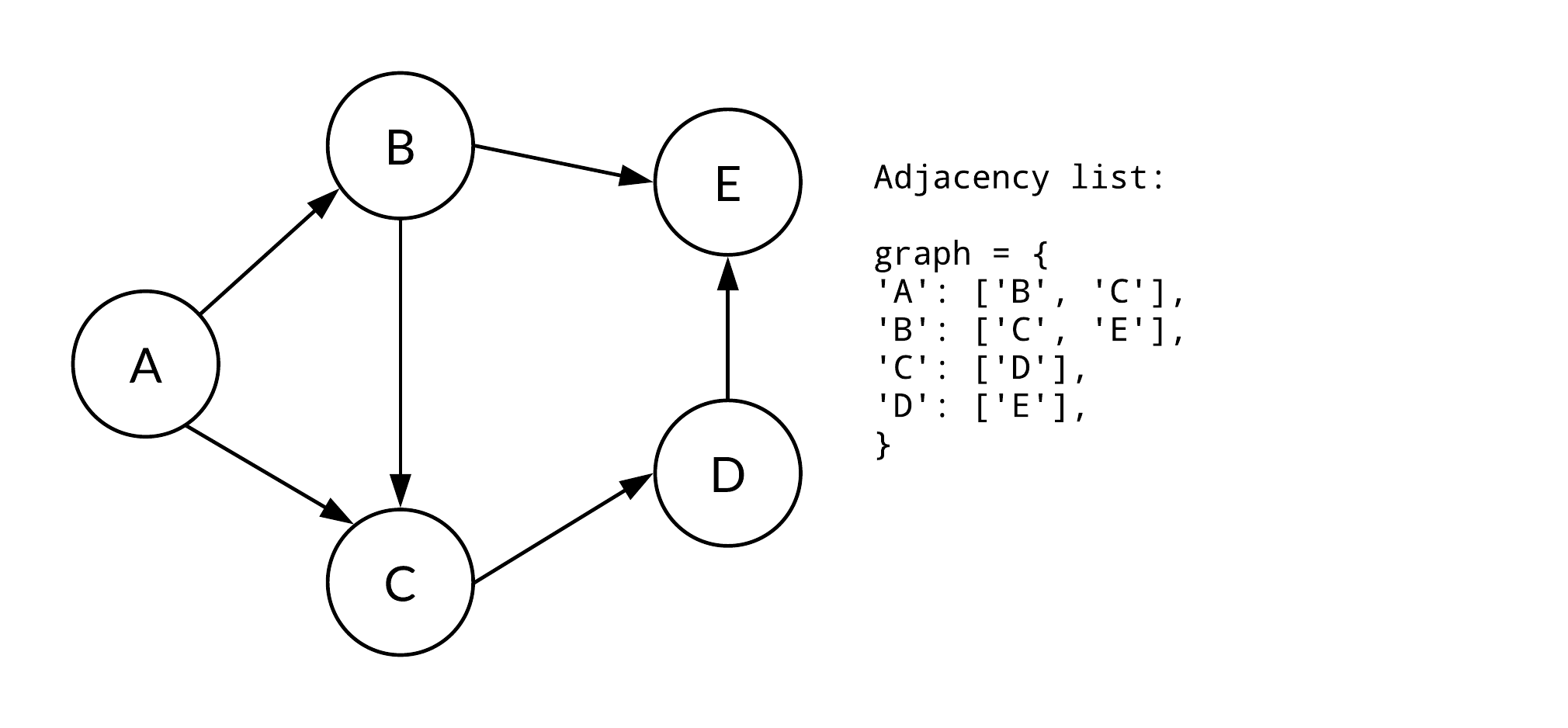Adjacency List — codepath

This first way is an adjacency list, this representation of a graph associate each vertex in the graph with the collection of its neighboring vertices or edges. The diagram above shows a javascript, directed adjacency list.

As an example of a textual relationship:

`NodeA: NodeB, NodeCNodeB: NodeC, NodeENodeC: NodeDNodeD: NodeE`

# Adjacency Matrix

The second way is an Adjacency Matrix (also called the connection matrix), which is a two-dimensional array, where each nested array has the same number of elements as the outer array. You can also say that it is a matrix that contains rows and columns that represent a labeled graph of the vertices with 0,1 in the position of (Vi, Vj), according to the conditions whether Vi and Vj are adjacent or not.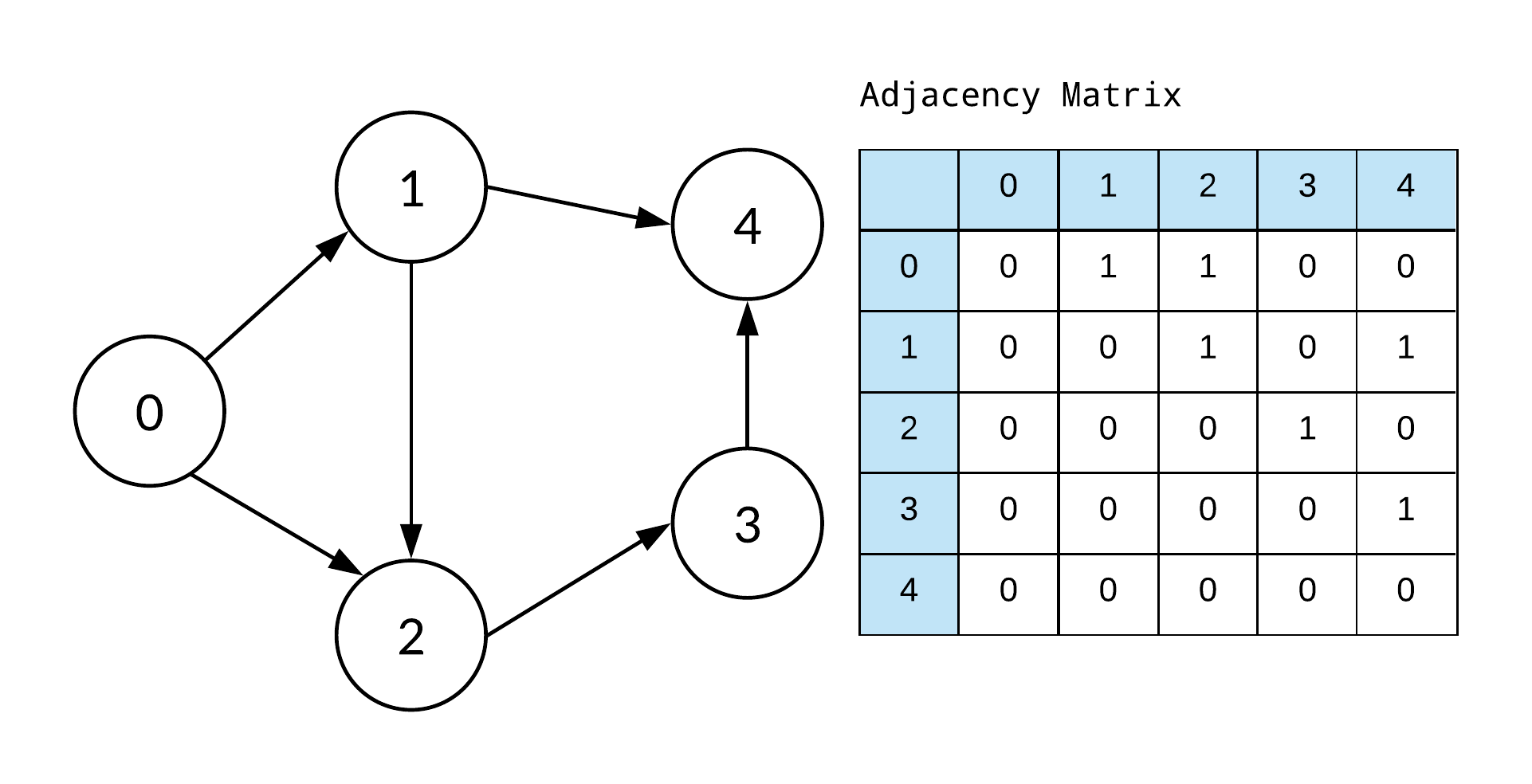Adjacency Matrix -Codepath

To better understand this diagram we ask:

• Is Vi (row) adjacent to Vj (column)?
• if it is an edge? it gets a 1
• Otherwise, it gets a zero

An example in javascript would be:

`var adjMat = [[0,1,1,0,0],[0,0,1,0,1],[0,0,0,1,0],[0,0,0, 0,1],[0,0,0,0,0]]`

Starting with the first row at 0, we ask “Is node0 adjacent to node0?” which of course it is not, so we put a 0. We then move on to ask is Node0 adjacent to node1, node2, node3,, etc and go along adding 1 or 0 into the array. Then move on to the next node and ask the same thing.

# Incidence Matrix

The third way is an Incidence Matrix, which is also a two-dimensional array. However, the incidence matrix uses rows to represent vertices (or nodes)and columns represent edges (or lines).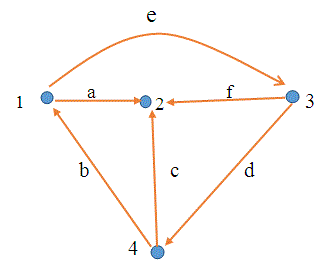Incidence Matrix with 4 nodes and 6 edges — Electrical4U

Below we have the incidence matrix for the graph above:

For the directed graph above, there are three things going on here:

• When a node has an outgoing edge, we write 1
• When a node has an incoming edge, we write -1
• When neither is happen for that node, you write 0

Here is the javascript instance of an incidence matrix:

`var incMatrx = [[1,-1,0,0,1,0],[-1,0,-1,0,0,-1],[0,0,01,-1,1],[0,1,1,-1,0,0]]`

To break it down, starting with Node1, we see that edgeA is outgoing so we add a 1 to the array, we noticed that edgeB is incoming so we write -1 in the array. However, for edgeC and edgeD, it looks like there are no relationships between them and Node1, so we can write 0 to the array.